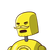# 4.a)In how many years will Rs. 950 produce Rs. 399 as simple interest at 7%?

4.a)In how many years will Rs. 950 produce Rs. 399 as simple interest at 7%?

### 1 thought on “4.a)In how many years will Rs. 950 produce Rs. 399 as simple interest at 7%?”

1.6 years

Thus, in 6 years will ₹ 950 produce ₹399 as simple interest at 7%.

Correct option is

A

6

Simple Interest I=100PTR

Given,

I=Rs399

P=Rs950

R=7%

So, 399=100950×T×7

=>T=6 years